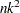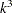Input Data Sets

PROC REG does not compute new regressors. For example, if you want a quadratic term in your model, you should create a new variable when you prepare the input data. For example, the statement

```model y=x1 x1*x1;
```

is not valid. Note that this MODEL statement is valid in the GLM procedure.

The input data set for most applications of PROC REG contains standard rectangular data, but special TYPE=CORR, TYPE=COV, and TYPE=SSCP data sets can also be used. TYPE=CORR and TYPE=COV data sets created by the CORR procedure contain means and standard deviations. In addition, TYPE=CORR data sets contain correlations and TYPE=COV data sets contain covariances. TYPE=SSCP data sets created in previous runs of PROC REG that used the OUTSSCP= option contain the sums of squares and crossproducts of the variables.

See Appendix A, Special SAS Data Sets, and the "SAS Files" section in SAS Language Reference: Concepts for more information about special SAS data sets.

These summary files save CPU time. It takesoperations (where=number of observations and=number of variables) to calculate crossproducts; the regressions are of the order. Whenis in the thousands andis less than, you can save 99% of the CPU time by reusing the SSCP matrix rather than recomputing it.

When you want to use a special SAS data set as input, PROC REG must determine the TYPE for the data set. PROC CORR and PROC REG automatically set the type for their output data sets. However, if you create the data set by some other means (such as a DATA step), you must specify its type with the TYPE= data set option. If the TYPE for the data set is not specified when the data set is created, you can specify TYPE= as a data set option in the DATA= option in the PROC REG statement. For example:

```proc reg data=a(type=corr);
```

When a TYPE=CORR, TYPE=COV, or TYPE=SSCP data set is used with PROC REG, statements and options that require the original data values have no effect. The OUTPUT, PAINT, PLOT, and REWEIGHT statements and the MODEL and PRINT statement options P, R, CLM, CLI, DW, INFLUENCE, and PARTIAL are disabled since the original observations needed to calculate predicted and residual values are not present.

### Example Using TYPE=CORR Data Set

The following statements use PROC CORR to produce an input data set for PROC REG. The fitness data for this analysis can be found in Example 76.2.

```proc corr data=fitness outp=r noprint;
var Oxygen RunTime Age Weight RunPulse MaxPulse RestPulse;
proc print data=r;
proc reg data=r;
model Oxygen=RunTime Age Weight;
run;
```

Since the OUTP= data set from PROC CORR is automatically set to TYPE=CORR, the TYPE= data set option is not required in this example. The data set containing the correlation matrix is displayed by the PRINT procedure as shown in Figure 76.14. Figure 76.15 shows results from the regression that uses the TYPE=CORR data as an input data set.

Figure 76.14 TYPE=CORR Data Set Created by PROC CORR
Obs _TYPE_ _NAME_ Oxygen RunTime Age Weight RunPulse MaxPulse RestPulse
1 MEAN   47.3758 10.5861 47.6774 77.4445 169.645 173.774 53.4516
2 STD   5.3272 1.3874 5.2114 8.3286 10.252 9.164 7.6194
3 N   31.0000 31.0000 31.0000 31.0000 31.000 31.000 31.0000
4 CORR Oxygen 1.0000 -0.8622 -0.3046 -0.1628 -0.398 -0.237 -0.3994
5 CORR RunTime -0.8622 1.0000 0.1887 0.1435 0.314 0.226 0.4504
6 CORR Age -0.3046 0.1887 1.0000 -0.2335 -0.338 -0.433 -0.1641
7 CORR Weight -0.1628 0.1435 -0.2335 1.0000 0.182 0.249 0.0440
8 CORR RunPulse -0.3980 0.3136 -0.3379 0.1815 1.000 0.930 0.3525
9 CORR MaxPulse -0.2367 0.2261 -0.4329 0.2494 0.930 1.000 0.3051
10 CORR RestPulse -0.3994 0.4504 -0.1641 0.0440 0.352 0.305 1.0000

Figure 76.15 Regression on TYPE=CORR Data Set
The REG Procedure
Model: MODEL1
Dependent Variable: Oxygen

Analysis of Variance
Source DF Sum of
Squares
Mean
Square
F Value Pr > F
Model 3 656.27095 218.75698 30.27 <.0001
Error 27 195.11060 7.22632
Corrected Total 30 851.38154

 Root MSE R-Square 2.68818 0.7708 47.3758 0.7454 5.67416

Parameter Estimates
Variable DF Parameter
Estimate
Standard
Error
t Value Pr > |t|
Intercept 1 93.12615 7.55916 12.32 <.0001
RunTime 1 -3.14039 0.36738 -8.55 <.0001
Age 1 -0.17388 0.09955 -1.75 0.0921
Weight 1 -0.05444 0.06181 -0.88 0.3862

The following example uses the saved crossproducts matrix:

```proc reg data=fitness outsscp=sscp noprint;
model Oxygen=RunTime Age Weight RunPulse MaxPulse RestPulse;
proc print data=sscp;
proc reg data=sscp;
model Oxygen=RunTime Age Weight;
run;
```

First, all variables are used to fit the data and create the SSCP data set. Figure 76.16 shows the PROC PRINT display of the SSCP data set. The SSCP data set is then used as the input data set for PROC REG, and a reduced model is fit to the data.

Figure 76.16 TYPE=SSCP Data Set Created by PROC REG
Obs _TYPE_ _NAME_ Intercept RunTime Age Weight RunPulse MaxPulse RestPulse Oxygen
1 SSCP Intercept 31.00 328.17 1478.00 2400.78 5259.00 5387.00 1657.00 1468.65
2 SSCP RunTime 328.17 3531.80 15687.24 25464.71 55806.29 57113.72 17684.05 15356.14
3 SSCP Age 1478.00 15687.24 71282.00 114158.90 250194.00 256218.00 78806.00 69767.75
4 SSCP Weight 2400.78 25464.71 114158.90 188008.20 407745.67 417764.62 128409.28 113522.26
5 SSCP RunPulse 5259.00 55806.29 250194.00 407745.67 895317.00 916499.00 281928.00 248497.31
6 SSCP MaxPulse 5387.00 57113.72 256218.00 417764.62 916499.00 938641.00 288583.00 254866.75
7 SSCP RestPulse 1657.00 17684.05 78806.00 128409.28 281928.00 288583.00 90311.00 78015.41
8 SSCP Oxygen 1468.65 15356.14 69767.75 113522.26 248497.31 254866.75 78015.41 70429.86
9 N   31.00 31.00 31.00 31.00 31.00 31.00 31.00 31.00

Figure 76.17 also shows the PROC REG results for the reduced model. (For the PROC REG results for the full model, see Figure 76.29.)

Figure 76.17 Regression on TYPE=SSCP Data Set
The REG Procedure
Model: MODEL1
Dependent Variable: Oxygen

Analysis of Variance
Source DF Sum of
Squares
Mean
Square
F Value Pr > F
Model 3 656.27095 218.75698 30.27 <.0001
Error 27 195.11060 7.22632
Corrected Total 30 851.38154

 Root MSE R-Square 2.68818 0.7708 47.3758 0.7454 5.67416

Parameter Estimates
Variable DF Parameter
Estimate
Standard
Error
t Value Pr > |t|
Intercept 1 93.12615 7.55916 12.32 <.0001
RunTime 1 -3.14039 0.36738 -8.55 <.0001
Age 1 -0.17388 0.09955 -1.75 0.0921
Weight 1 -0.05444 0.06181 -0.88 0.3862

In the preceding example, the TYPE= data set option is not required since PROC REG sets the OUTSSCP= data set to TYPE=SSCP.